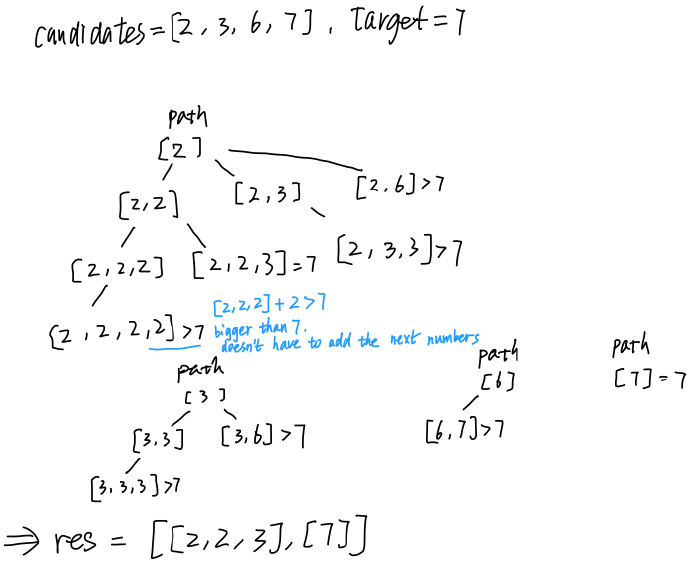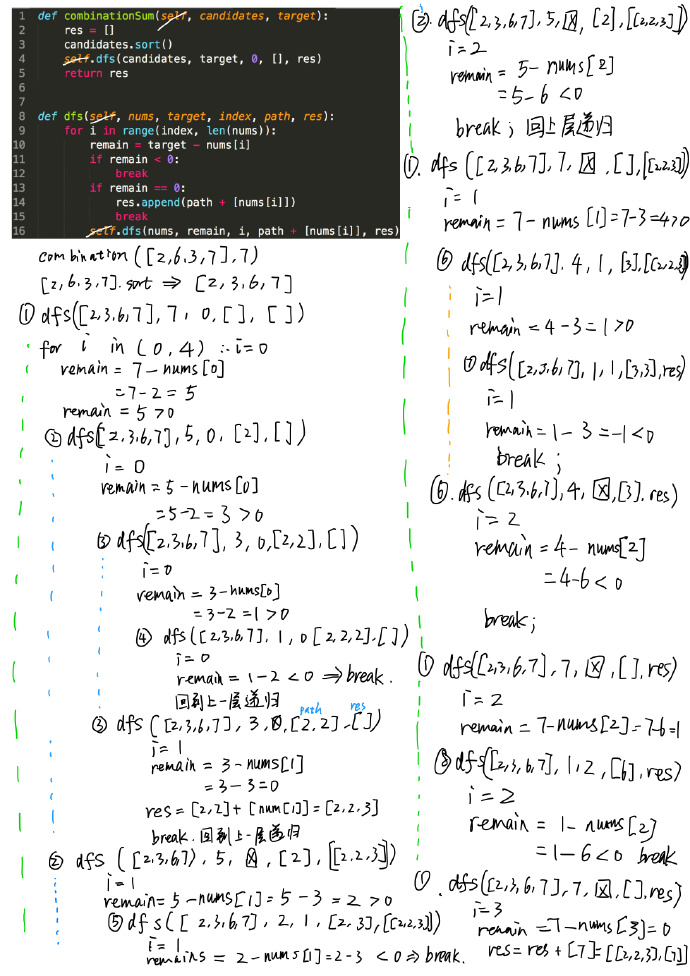# LeetCode 39 Combination Sum

## Description

https://leetcode.com/problems/combination-sum/

Given a set of candidate numbers (candidates) (without duplicates) and a target number (target), find all unique combinations in candidates where the candidate numbers sums to target.

The same repeated number may be chosen from candidates unlimited number of times.

Note:

All numbers (including target) will be positive integers.
The solution set must not contain duplicate combinations.

Example 1:

``````Input: candidates = [2,3,6,7], target = 7,
A solution set is:
[
,
[2,2,3]
]
``````

Example 2:

``````Input: candidates = [2,3,5], target = 8,
A solution set is:
[
[2,2,2,2],
[2,3,3],
[3,5]
]
``````

## Solution

### Expalnation:

• Sort the list at first, so once the remain less than 0, it doesn’t have to add next numbers of the list.
• The process of addition likes deep first sort.### Full Code in Github :

https://github.com/younglaker/leetcode/blob/master/39_combination_sum.py

### Code explanationExchange blogroll： http://laker.me/blog
Github：https://github.com/younglaker

LeetCode 39 Combination Sum